Type 1 pseudo amino acid composition
Type 1 PseAA composition is also called the parallel-correlation type and generates 20 +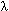discrete numbers to represent a protein, which was introduced by Prof. Kuo-Chen Chou in 2001 and the original publication is:

Chou K.C.(2001). Prediction of protein cellular attributes using pseudo-amino-acid-composition. PROTEINS: Structure, Function, and Genetics 43, 246-255.

The essence of pseudo-amino acid composition is, on one hand, to include the main feature of amino acid composition, but on the other, to include information beyond amino acid composition. The conventional amino acid composition contains 20 components, or discrete numbers, each reflecting the occurrence frequency of one of the 20 native amino acids in a protein. For the pseudo-amino acid composition, however, there are some other elements in addition to the 20 components. It is through these additional discrete numbers that the sequence order effect of a protein is approximately reflected and improvements are made, as will be shown below. The basic ideas of Type 1 pseudo amino acid composition is as following:
Consider a protein chain of L amino acid residues: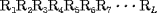(1)
Sequence order effect can be approximately reflected with a set of sequence order-correlated factors as defined below: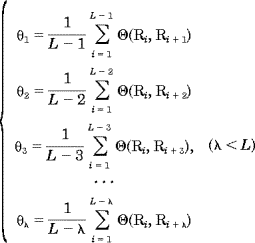(2)
where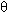1 is called the first-tier correlation factor that reflects the sequence order correlation between all the most contiguous residues along a protein chain (Fig. 1a),2 the second-tier correlation factor that reflects the sequence order correlation between all the second most contiguous residues (Fig.1b),3 the third-tier correlation factor that reflects the sequence order correlation between all the 3rd most contiguous residues (Fig.1c), and so forth. In Eq. 2 the correlation function is given by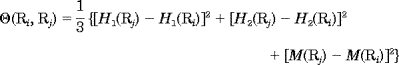(3)
where H1(Ri), H2(Ri), and M(Ri) are, respectively, the hydrophobicity value, hydrophilicity value, and side-chain mass of the amino acid Ri; and H1(Rj), H2(Rj), and M(Rj) the corresponding values for the amino acid Rj. Note that before substituting the values of hydophobicity, hydrphilicity, and side-chain mass into Eq. 3, they were all subjected to a standard conversion as described by the following equation: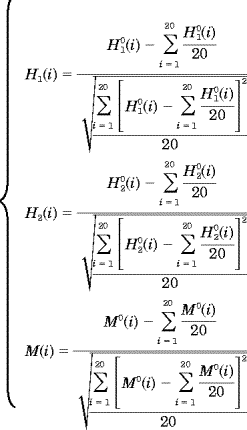(4)
where H(i) is the original hydrophobicity value of the ith amino acid, H(i) the corresponding original hydrophilicity value, and M0(i) the mass of the ith amino acid side chain that can be obtained from any biochemistry text book. Without loss of generality, we use the numerical indices 1, 2, 3,, 20 to represent the 20 native amino acids according to the alphabetical order of their single-letter codes: A, C, D, E, F, G, H, I, K, L, M, N, P, Q, R, S, T, V, W, and Y. The data obtained by such a standard conversion (Eq. 4) will have a zero mean value and will remain unchanged if going through the same conversion procedure again.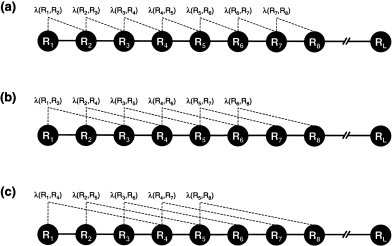Figure 1. A schematic drawing to show (a) the first-tier, (b) the second-tier, and (3) the third-tier sequence order correlation mode along a protein sequence. Panel (a) reflects the correlation mode between all the most contiguous residues, panel (b) that between all the secon-most contiguous residues, and panel (c) that between all the third-most contiguous residues.
As we can see from Figure 1, the sequence order effect of a protein can be, to some extent, reflected through a set of sequence-correlation factors1,2,3,,, as defined by Eq. 2. Now let us augment the formulation of amino acid composition to include such a set of discrete numbers. To realize this, instead of using a 20-D (dimensional) vector defined by 20 components, we use a (20 +)-D vector defined by 20 +discrete numbers to represent a protein X; i.e.,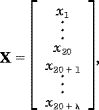(5)
where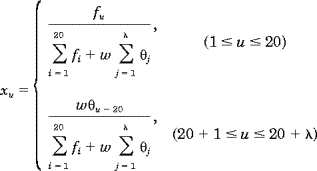(6)
where fi is the normalized occurrence frequency of the 20 amino acids in the protein X,j is the j-tier sequence correlation factor computed according to Eqs. 2-4 for the protein X, and w is the weight factor for the sequence order effect. As we can see from Eqs. 5 and 6, the first 20 components reflect the effect of the amino acid composition, whereas the components from 20 + 1 to 20 +reflect the effect of sequence order. A set of such 20 +components as formulated by Eqs. 5 and 6 is called the pseudo-amino acid composition for protein X.